Plotting exercisesWeiran Huang Nov 17, 2018

My personal implementation for the exercises for chapter 6 in the book scientific computing with Python3.

from scipy import *
from matplotlib.pyplot import *

%matplotlib inline


# Before we start

All the solutions are given by Waiyin Wong, to whom the copyright belongs.

There are 6 exercises in total, but because of the final examinations, this article have to be updated later.

Update: I’ve finished all the 6 exercises on Jan 23rd, 2018.

# Plotting exercises

Ex. 1 Write a function that plots an ellipse given its center coordinates $(x,y)$ , the half axis $a$ and $b$ rotation angle $\theta$.

Here we assume $\theta$ is the degree of angle that we rotate the standard ellipse, in order to get our one.(let’s say, counter-clockwise)

In other word, there is a linear mapping between the coordinates after rotation $(x’,y’)$ and the original $(x,y)$:

$$\left[\begin{matrix}x’ \\ y’\end{matrix}\right] = \left[\begin{matrix}cos\theta & -sin\theta \\ sin\theta & cos\theta\end{matrix}\right]\left[\begin{matrix}x \\ y\end{matrix}\right]$$

or, in another form:

$$\left[\begin{matrix}x \\ y\end{matrix}\right] = \left[\begin{matrix}cos\theta & sin\theta \\ -sin\theta & cos\theta\end{matrix}\right]\left[\begin{matrix}x’ \\ y’\end{matrix}\right]$$

where $x,y$ satisfy：
$$\frac{x^2}{a^2} + \frac{y^2}{b^2} = 1$$

def draw_ellipse(a, b, x0, y0, theta):
'''
to draw a ellipse given the half axis a,b and the rotation angle theta.
'''
x,y = meshgrid(linspace(-a-1, a+1, 100), linspace(-a-1,a+1, 100))
contour(x*cos(theta)-y*sin(theta) + x0, x*sin(theta)+y*cos(theta) + y0, x**2/a**2 + y**2/b**2 - 1, )

draw_ellipse(3,2,1,2,pi/3)
title('an example ellipse')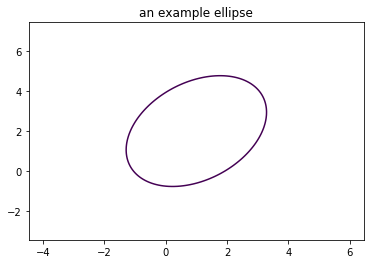There is another way to draw an ellipse except using the command contour.

Consider the parametric equation of an ellipse(standard form):

$$\begin{equation} \begin{cases} x = a \cdot cos\alpha\\ y = b \cdot sin\alpha \end{cases} \end{equation}$$

The rotation can be done the same way as above.

So we can do as below:

def draw_ellipse2(a, b, x0, y0, theta):
'''
to draw a ellipse given the half axis a,b and the rotation angle theta.
'''
alpha = linspace(0, 2*pi, 200)
x = a * cos(alpha)
y = b * sin(alpha)
x1 = x*cos(theta)-y*sin(theta)
y1 = x*sin(theta)+y*cos(theta)

plot(x1 + x0, y1 + y0)
axis([-12,12,-12,12])  # set the axis

draw_ellipse2(9, 4, 1, 2, pi/3)
draw_ellipse2(4, 2, -1, 0,pi/2)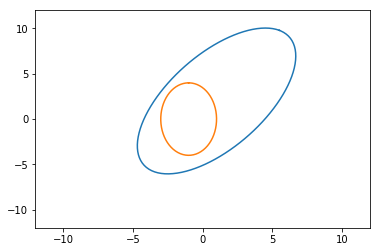Ex. 2 Write a short program that takes a 2D array, e.g., the preceding Mandelbrot contour image, and iteratively replace each value by the average of its neighbors. Update a contour plot of the array in a figure window to animate the evolution of the contours. Explain the behavior.

We’ve mentioned how to use the command imshow to draw the Mandelbrot fractal in this article. Now we need only to change a bit our former code by introduce the command contour instead.

def mandelbrot(h,w, maxit=20):
X,Y = meshgrid(linspace(-2, 0.8, w), linspace(-1.4, 1.4, h))
c = X + Y*1j
z = c
exceeds = zeros(z.shape, dtype=bool)

for iteration in range(maxit):
z = z**2 + c
exceeded = abs(z) > 4
exceeds_now = exceeded & (logical_not(exceeds))
exceeds[exceeds_now] = True
z[exceeded] = 2 # limit the values to avoid overflow

return exceeds

figure(figsize = (8,8))
#imshow(mandelbrot(400,400),cmap='gray')
#contour(mandelbrot(400,400), levels=logspace(-4.7, 3., 10), colors='black', alpha=0.5)
contour(mandelbrot(400,400), colors = 'black')
axis('off')

(0.0, 399.0, 0.0, 399.0)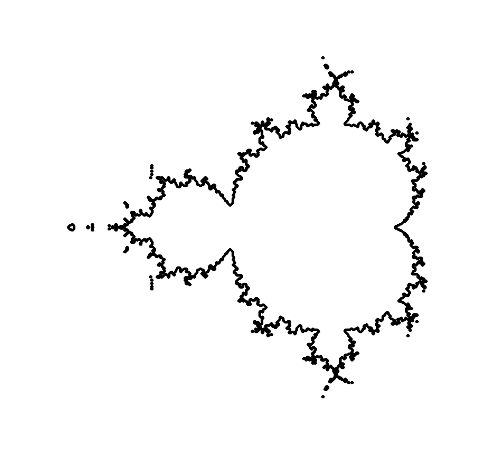Then let’s see back to the questions.

you should remember the code below:

def avg(x):
""" simple running average """
return (roll(x,1) + x + roll(x,-1)) / 3

# sine function with noise
x = linspace(-2*pi, 2*pi,200)
y = sin(x) + 0.4*rand(200)

# make successive subplots
for iteration in range(3):
subplot(3, 1, iteration + 1)
plot(x,y, label = '{:d} average{}'.format(iteration, 's' if iteration > 1 else ''))
yticks([])
legend(loc = 'lower left', frameon = False)
y = avg(y) #apply running average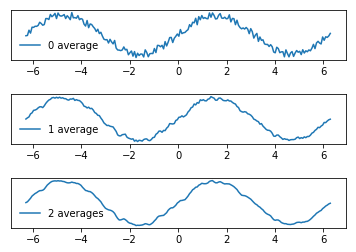We can adopt the same method in this case:

def avg_2d(x):
'''
running average on a 2D array
'''
return (roll(x,1,axis = 0) + roll(x,-1,axis = 0) + x + roll(x,1,axis = 1) + roll(x,-1, axis = 1))/5

x = mandelbrot(400, 400)

figure(figsize = (8,32))
for iteration in range(4):
subplot(4, 1, iteration + 1)
title('{:d} average{}'.format(iteration, 's' if iteration > 1 else ''))
x = avg_2d(x) # apply running average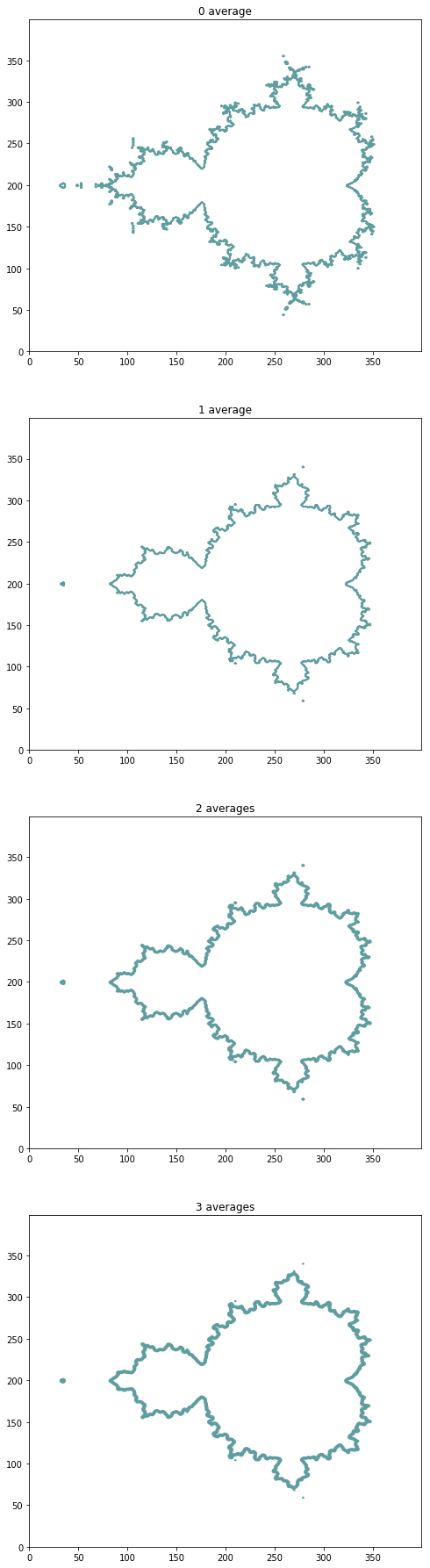Ex. 3 Consider an $N \times N$ matrix or image with integer values. The mapping

$$I:(x,y) \mapsto (2x+y, x+y)\space mod\space N$$

is an example of a mapping of a toroidal square grid of points onto itself. This has the interesting property that it distorts the image by shearing and then moving the pieces outside the image back using the modulu function mod. Applied iteratively, this results in randomizing the image in a way that eventually returns the original. Implement the following sequence:

$$I^{(m+1)}(x,y) =I^{(m)}(2x+y\space mod\space N, x+y\space mod\space N)$$

and save out the first $N$ steps to files or plot them in a figure window.
As an example image, you can use the classic $512 × 512$ Lena test image from scipy.misc.

from scipy.misc import lena
I = lena()


The result should look like this:As the example shows we need to deal with an image. But unfortunately we cannot use the example image lena, because it has been removed from scipy.misc for liscence reason.

We decide to use the image ascent instead as below.

from scipy.misc import ascent
I = ascent()

imshow(I)
I.shape

(512, 512)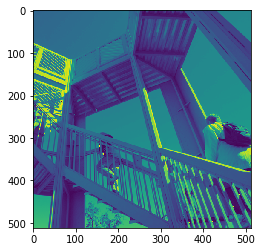But to be honest, I could not really understand this question.
I cannot see the point why the image will return to the original. And I think for a $512\times 512$ image, 512 steps is (if it could) not enough to return. So I simply plot the 6 steps here.

def distort_img(x_range, y_range, I,plt_loc):
subplot(1,10, plt_loc)
imshow(I)
temp = I
for x in range(x_range):
for y in range(y_range):
temp[x,y] = I[(2*x+y)%x_range, (x+y)%y_range]
I = temp

axis('off')

fig = figure(figsize = (20,10))
#subplot(1,5,1)
#imshow(I)

x_range, y_range = I.shape
for iteration in range(9):
distort_img(x_range, y_range, I, iteration + 1)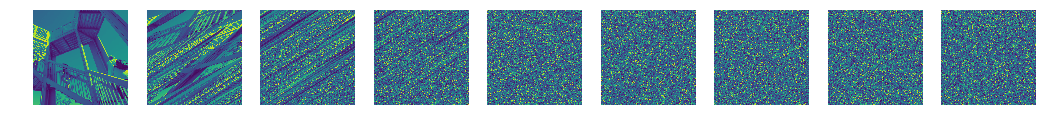Ex. 4 Reading and plotting on images. SciPy comes with the imread function (in the scipy.misc module) for reading images, (refer to section Reading and Writing Images in Chapter 12, Input and output). Write a short program that reads an image from file and plots the image contour at a given gray level value overlaid on the original image.

Tips: You can get a gray level version of the image by averaging the color
channels like this: mean(im,axis=2)
As below:

from scipy.misc import face
figure()
subplot(121)
m = face()
imshow(m)
n = mean(m, axis = 2)
subplot(122)
imshow(n)

<matplotlib.image.AxesImage at 0x2609cc19518>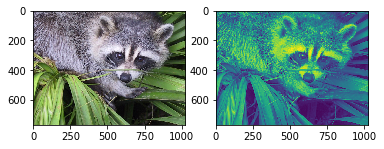However, you may find one problem that you cannot use the function imread because you did not install the Python imaging library. If you are a windows user, you cannot download it using the command pip. But we can pip its subset pillow instead.

# Install a pip package in the current Jupyter kernel
import sys
!{sys.executable} -m pip install pillow

Requirement already satisfied: pillow in c:\users\huang\pycharmprojects\review_python\venv\lib\site-packages


Then We start to deal with this problem. I chose a pic of Messi and downloaded locally. After that I use imread and imshow to display it.

from scipy.misc import imread

im_gray = mean(im, axis = 2)
figure(figsize = (20,20))
subplot(121)
imshow(im)

subplot(122)
imshow(im_gray)
contour(im_gray)

axis('off')

c:\users\huang\pycharmprojects\review_python\venv\lib\site-packages\ipykernel_launcher.py:3: DeprecationWarning: imread is deprecated!
imread is deprecated in SciPy 1.0.0, and will be removed in 1.2.0.
Use imageio.imread instead.
This is separate from the ipykernel package so we can avoid doing imports until

(-0.5, 1279.5, 719.5, -0.5)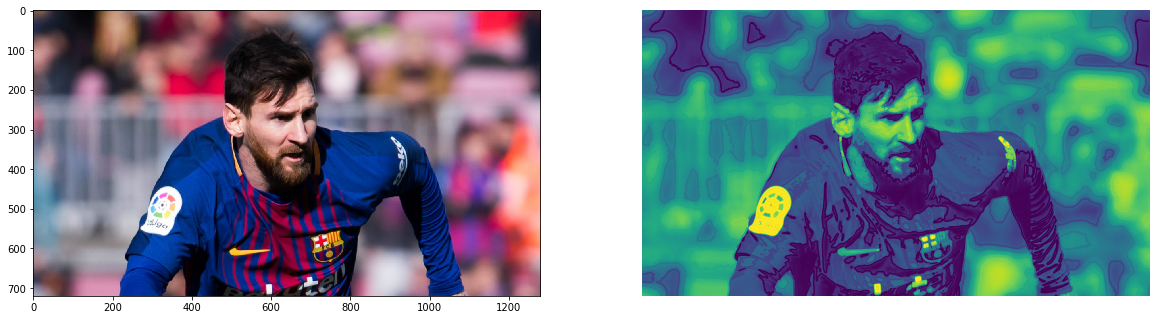But as is showed in the warning, we know that the function imread is going to be removed from scipy.misc:

DeprecationWarning: imread is deprecated!
imread is deprecated in SciPy 1.0.0, and will be removed in 1.2.0.
Use imageio.imread instead.
This is separate from the ipykernel package so we can avoid doing imports until

We can use imageio.imread instead.

Ex. 5 Image edges. The zero crossings of the 2D Laplacian are a good indication of image
edges. Modify the program in the previous exercise to use the gaussian_laplace or
laplace function from the scipy.ndimage module to compute the 2D Laplacian and
overlay the edges on top of the image.

from scipy import ndimage,misc
import PIL
k = im_gray
#gray()
result = ndimage.gaussian_laplace(k,sigma = 3)

gray()
figure(figsize = (20,20))
subplot(121)
imshow(k)

# put the two images together to see the difference
subplot(122)
imshow(k)
# using the transparency parameter alpha to overlay an image on another
imshow(result, alpha = 0.5)

<matplotlib.image.AxesImage at 0x250acc04898>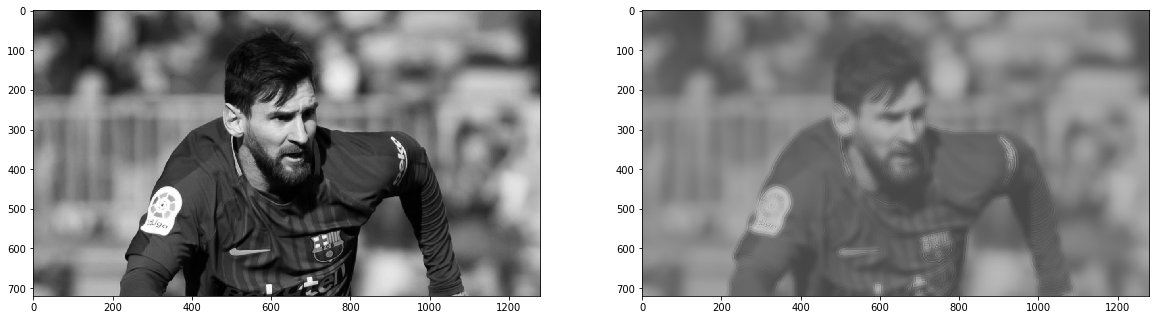Ex. 6 Reformulate the Mandelbrot fractal example (see section Images and Contours) by
using orgid instead of meshgrid, see also the explanation ogrid in Function of two variables
in Chapter 5, Advanced Array Concepts. What is the difference between orgid, mgrid, and
meshgrid?

As the question indicating, we have learned something about the class(note: not a function) ogrid in previous chapter.
It can be used to generate tuples for broadcasting, as the example shows below:

x,y = ogrid[0:1:3j, 0:1:3j]
print(x,y)
print('\n x + y is')
print(x+y)

[[ 0. ]
[ 0.5]
[ 1. ]] [[ 0.   0.5  1. ]]

x + y is
[[ 0.   0.5  1. ]
[ 0.5  1.   1.5]
[ 1.   1.5  2. ]]


And as for meshgrid, we know that such an expression

X,Y = meshgrid(linspace(a,b,m),linspace(c,d,n))


X,Y will are arrays with (m,n) shapes such that $X[i][j]$ and $Y[i][j]$ contains the coordinates of the grid point $P[i][j]$.

def mandelbrot(h,w, maxit=20):
#X,Y = meshgrid(linspace(-2, 0.8, w), linspace(-1.4, 1.4, h))
Y,X = ogrid[-1.4:1.4:h*1j,-2:.8:w*1j] # since the first one is a column vector while the second a row vector
c = X + Y*1j
z = c
exceeds = zeros(z.shape, dtype=bool)

for iteration in range(maxit):
z = z**2 + c
exceeded = abs(z) > 4
exceeds_now = exceeded & (logical_not(exceeds))
exceeds[exceeds_now] = True
z[exceeded] = 2 # limit the values to avoid overflow

return exceeds

imshow(mandelbrot(400,400),cmap='gray')
axis('off')

(-0.5, 399.5, 399.5, -0.5)We can easily tell the difference between meshgrid and ogrid.
meshgrid generates two matrix, while ogrid generates one column vector and one row vector, and use broadcasting to generate a matrix. You may find meshgrid a little bit superfluous here. Actually it it just an implementation of MATLAB’s meshgrid, probably to cater to users coming from a MATLAB background.

We notice that the question also mentioned the mgrid. So let’s compare the behavior of mgrid and meshgrid.

x1, y1 = meshgrid(linspace(1,5,5), linspace(1,3,3))
print(x1) # a 3*5 array
print()
print(y1) # a 3*5 array

[[ 1.  2.  3.  4.  5.]
[ 1.  2.  3.  4.  5.]
[ 1.  2.  3.  4.  5.]]

[[ 1.  1.  1.  1.  1.]
[ 2.  2.  2.  2.  2.]
[ 3.  3.  3.  3.  3.]]

x2, y2 = mgrid[1:5:5j,1:3:3j]
print(x2) # a 5*3 array
print()
print(y2) # a 5*3 array

[[ 1.  1.  1.]
[ 2.  2.  2.]
[ 3.  3.  3.]
[ 4.  4.  4.]
[ 5.  5.  5.]]

[[ 1.  2.  3.]
[ 1.  2.  3.]
[ 1.  2.  3.]
[ 1.  2.  3.]
[ 1.  2.  3.]]


It returns just the transpose of the meshgrid`.

This blog is under a CC BY-NC-SA 3.0 Unported License